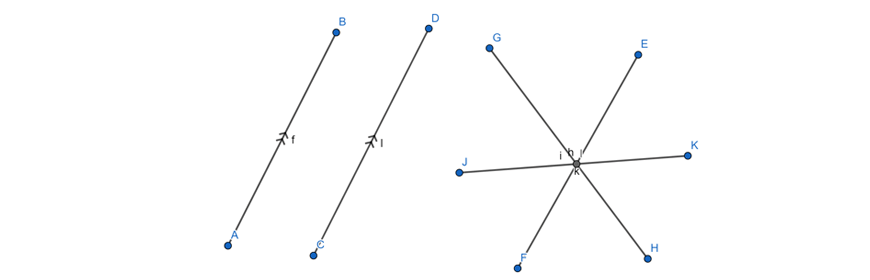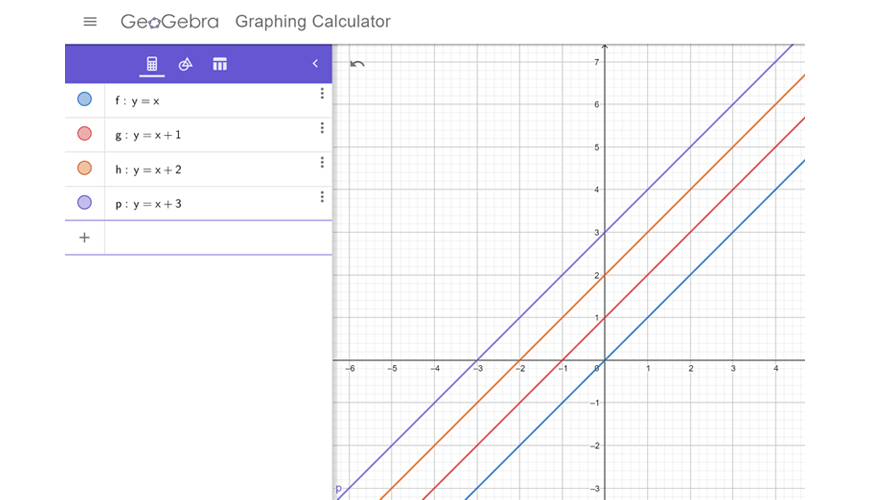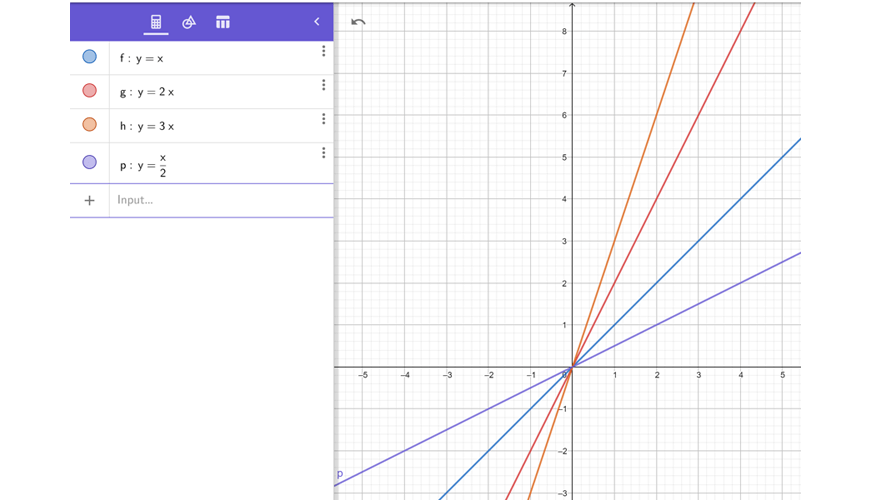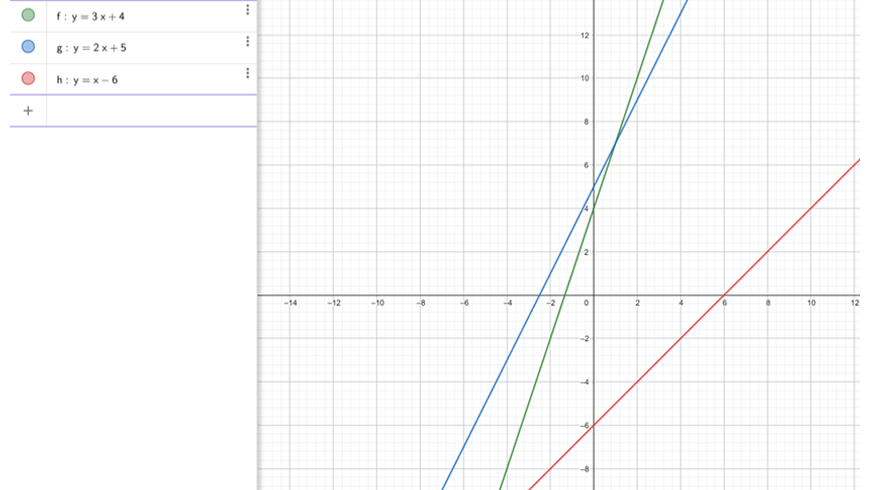# Part 3: Properties of Linear Relations

Are you unsure of what the properties of linear relations are? Don't fret! In this guide, we will explain the properties of linear relations (eg. what m means in y = mx +b), show you how to graph more than one line the same axes and illustrate how each term in the y = mx + b equation affect the line.## Properties of Linear Relations

In everyday life, many systems can be modelled by mathematical relationships.

Sometimes, more than one system may work to achieve the same task, or two systems may operate in similar ways, allowing us to compare and contrast them.

By considering the properties of relationships including linear relationships, we can make more informed decisions about these systems.

## NSW Syllabus outcomes

 Syllabus Explanation NSW Stage 4 NESA Syllabus Given coordinates, plot points on the Cartesian plane, and find coordinates for a given point (ACMNA178) Graph more than one line on the same set of axes using digital technologies and compare the graphs to determine similarities and differences, e.g. parallel, passing through the same pointDetermine which term of the rule affects the gradient of a graph, making it increase or decrease## Assumed knowledge

Students should be aware of how to plot a given line on a cartesian plane by hand.

## Properties of Linear Relations

Any linear relationship on a graph can be characterised by just two numbers:

• Intercept

Upon closer inspection, we can also find similarities between different lines.

When writing a linear relationship, we use the form $$y = mx + b$$ to concisely sum up the relationship.

• $$m$$ is the gradient
• $$b$$ is the intercept of the equation.

There are a few tools that we can use to visualise lines digitally, like GEOGEBRA.

To examine the effect of changing  $$m$$ and $$b$$, we can consider the following lines:When $$b$$ is changed, the line moves up and down.

If we take a look at the $$y-axis$$, the value of $$b$$ corresponds to the value of the intersection of the line and the $$y-axis$$.

Hence, $$b$$ is known as the $$y-intercept$$.

You may also notice that the lines in this diagram are parallel.

This is because we have left the steepness of the line $$(m)$$ unchanged.

What happens if we try and change the value of $$m$$?When $$m$$ is larger, the line becomes steeper; and when $$m$$ is smaller, the line becomes flatter.

$$m$$ is known as the gradient.

## Checkpoint question

1) Without drawing the 3 lines below, determine which one:

\begin{align*}
y = 3x + 4 , \ y = 2x + 5, \ y = x – 6
\end{align*}

a. Is the steepest

b. Is the least steep

c. Has the highest value when

d. Has the highest value when

2) Now, draw the following lines on the same set of axes on a sheet of paper.

## Solutions

1)

a. $$y = 3x + 4$$

b. $$y = x – 6$$

c. $$y = 2x + 5$$

d. $$y = 3x + 4$$

2)© Matrix Education and www.matrix.edu.au, 2021. Unauthorised use and/or duplication of this material without express and written permission from this site’s author and/or owner is strictly prohibited. Excerpts and links may be used, provided that full and clear credit is given to Matrix Education and www.matrix.edu.au with appropriate and specific direction to the original content.